# We can learn a lot from a walk with real numbers

Questions on the digit expansion of mathematical constants, such as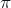,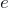, or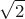have fascinated mathematicians for centuries. However, many of these questions remain elusive to the efforts of researchers.

For instance, it is not known for sure if the number 1 appears infinitely often in the decimal expansion of. Among the most remarkable questions is that of whether,, orare normal numbers.

A real number is said to be normal in base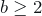if, in the expansion of the number in that base, every string of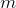digits in that base appears with the expected frequency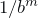, i.e., as if the expansion was randomly created.

Although seeming counterintuitive, it turns out that for a given base almost all real numbers are normal. Furthermore, almost all real numbers are simultaneously normal for every base(what is known as being absolutely normal).

Despite this fact, proving normality happens to be significantly difficult. There are proofs for some numbers, like the Champernowne number 0.12345678910111213141516… obtained by concatenation of naturals 1, or the Copeland-Erdős number 0.23571113171923… obtained by concatenation of primes 2, which are both normal in base 10.

But there are no proofs of normality for, among many others,,, or, although it is widely believed that they are normal and, actually, that every irrational algebraic number is absolutely normal.

Of course, computers have brought a powerful tool into this fight. The first computation ofup to 2037 decimal places on ENIAC in 1949, was proposed by John von Neumann in order to put some light on the distribution of the digits of, indeed 3.

As the computation power increased, more and more numbers in the expansions were known, so that techniques of scientific visualization and large-scale data analysis came into the game. This is the case of a recent and remarkable paper by Aragón Artacho et al. 4, in which the authors not only represent these data as walks in the plane, but also they make a rigorous and quantitative study of these walks.

First, they draw a uniform pseudo-random walk with one million base-4 steps, such that numbers 0, 1, 2, and 3 are translated into East, North, West, and South steps, respectively.

This walk is compared with that of the following rational numbers

which turn out to share the first 240 base-10 digits and the first 400 base-4 digits. It is not surprising, then, that their walks look similar, but it is quite surprising that they draw a blackboard bold letter Q.

In the first case the walk is bounded (using more steps leads to the same plot), while in the second case it unbounded but repeating a pattern after a certain number of digits.

Other rational numbers are not so easily identified by the plot of their walks. For example, one can construct 5 numbers having base-10 periodic parts of length 1,750,000,000 and 1,000,000,000,060 respectively, whose first million digits draw the following picture

(It is interesting to note that it is possible to create a rational number whose period is of any desired length.)

Then, the authors show a walk on the first 100 billion base-4 digits of, which can also be checked dynamically online 6.

After that, Aragón Artacho et al. show how the study of real numbers as walks allows to compare numbers which are known to be normal with others that are suspected to be so, as well as with random and pseudo-random numbers.

A first tool is the expected distance to the origin, which the authors prove to be asymptotically equal to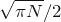for any base-b random walk of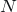steps. As a first example, the authors test a sample of 10,000 base-4 pseudo-random walks of one million steps, obtaining an average normalized distance to the origin of 1.0031 with a standard deviation of 0.3676. Then, they test 10,000 walks of one million steps taken from the first 10 billion digits of, obtaining an average normalized distance to the origin of 1.0008, with a standard deviation of 0.3682.

The authors collect results for several numbers in a table, with the striking surprise that, despite being normal, the average normalized distance to the origin for the Champernowne number is 59.91143, very far from the expected 1.

A second tool is the number of distinct points visited during a walk, whose expectation was proved to be asymptotically equal to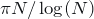for a random walk ofsteps on a two-dimensional lattice by Dvoretzky and Erdős 7. Since this asymptotic result has a rather slow convergence, the authors use instead lower and upper bounds by Downham and Fotopoulos 8.

They collect results in a table and, again, some numbers likefit quite well the expected bounds, while other numbers like base-4 Champernowne show substantial differences with the expectation.

Next, the authors describe and study concatenation numbers, a generalization of Champernowne and Copeland-Erdős numbers, as well as Stoneham numbers, defined 9 as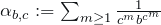for relatively prime integers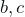.

In a final section, the authors explore other techniques like the fractal dimension (more specifically the box-counting or Minkowski-Bouligand dimension). They also plot a base-4 walk on a million bases of the X-chromosome and compare it with a million digits of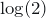.

Matrix representations, random chaos games, and turtle plots are also considered, for a deeper insight into different numbers.

Overall, this paper shows how mathematical research can deal with difficult questions using quite intuitive ideas and, on the way, producing beautiful pictures.

## References

1. David G. Champernowne. The Construction of Decimals Normal in the Scale of Ten. Journal of the London Mathematical Society s1-8:4 (1933) 254-260. http://dx.doi.org/10.1112/jlms/s1-8.4.254
2. Arthur H. Copeland and Paul Erdős. Note on normal numbers. Bulletin of the American Mathematical Society 52:10 (1946) 857-860. http://dx.doi.org/10.1090/S0002-9904-1946-08657-7
3. Lennart Berggren, Jonathan M. Borwein, and Peter B. Borwein. Pi: a Source Book. Springer-Verlag, Third Edition, 2004. http://dx.doi.org/10.1007/978-1-4757-2736-4
4. Aragón Artacho F.J., Bailey D.H., Borwein J.M. & Borwein P.B. (2013). Walking on Real Numbers, The Mathematical Intelligencer, 35 (1) 42-60. DOI: 10.1007/s00283-012-9340-x
5. George Marsaglia. On the randomness of pi and other decimal expansions. InterStat, October 2005. http://interstat.statjournals.net/YEAR/2005/abstracts/051005.php
6. http://gigapan.org/gigapans/106803 Last access: February 2014.
7. Aryeh Dvoretzky and Paul Erdős. Some Problems on Random Walk in Space. Proceedings of the 2nd Berkeley Symposium on Mathematical Statistics and Probability, (1951), 353–367. http://projecteuclid.org/euclid.bsmsp/1200500239
8. Stergios B. Fotopoulos. A note on the simple random walk in the plane. Statistics & Probability Letters 17:3 (1993) 221-224. http://dx.doi.org/10.1016/0167-7152(93)90170-N
9. David H. Bailey and Richard E. Crandall. Random Generators and Normal Numbers. Experimental Mathematics 11:4 (2002) 527-546. http://www.emis.de/journals/EM/expmath/volumes/11/11.4/pp527_546.pdf